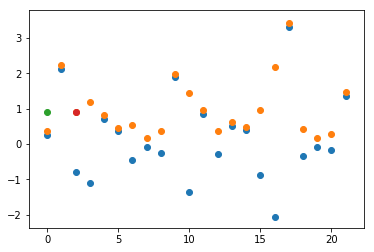PAMAdam (Parameter-wise Absolute Mean ADAM) is my new optimizer. It is very similar to LAMB, but it uses different function called absme:

def absme(t):
return t.abs().mean()


This absme will be applied on the parameter tensor and on the Adam step. Let’s explain what absme will do in a diagram:

t = torch.randn(22)
q = t.abs()
m = q.mean()

def absme(t):
return t.abs().mean()

plt.scatter(range(0,22), t) #blue
plt.scatter(range(0,22), q+0.1) #orange
plt.scatter(range(0,1), m) #green

a = absme(t)
plt.scatter(range(2,3), a) #redBlue dot’s are data from the normal distribution, orange dots are abs() of blues, green and red are same absme.

We created and named the function absme to express the abs, mean operation.

Inside PAMAdam at the very end we will calculate absme two times:

absme1 = absme(p.data)
absme2 = absme(step)


Where p.data is the parameter, and the step is the Adam step. Note we removed the weight decay entirely because it is not needed since we always use batch norms.

Then the final update of param p would be:

p.data = p.data - lr*absme1/absme2 * step


Here is the full code:

class PAMAdam(Optimizer):

def __init__(self, params, lr=1e-3, betas=(0.9, 0.999), eps=1e-8):

defaults = dict(lr=lr, betas=betas, eps=eps)

def __setstate__(self, state):

def absme(self,t):
return t.abs().mean()

def step(self, closure=None):

for group in self.param_groups:
for p in group['params']:

if p.grad is None:
continue

state = self.state[p]

if len(state) == 0:
state['step'] = 0

state['step'] += 1

beta1, beta2 = group['betas']

bias_1 = 1 - beta1 ** state['step']
bias_2 = 1 - beta2 ** state['step']